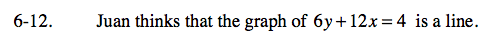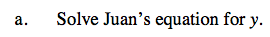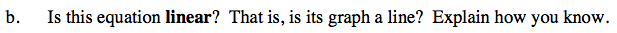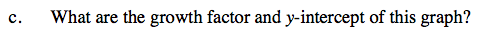### Home > CAAC > Chapter 6 > Lesson 6.1.1 > Problem6-12

6-12.Get y by itself by subtracting 12x from both sides.

$y= -2x + \frac{2}{3}$

6y + 12x = 4
−12x −12x
6y = 4 − 12xCan it be written in the form y = mx + b?What is m in this equation? What is b?